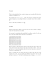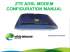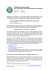## Transcription

```Adjusting the Asymmetric Two Sample t-Test
Sandy D. Balkin
Ernst & Young LLP
1225 Connecticut Avenue, NW
Washington, DC 20037
Abstract
The Telecommunications Act of 1996 requires that Incumbent Local Exchange Carriers (ILECs) must provide,
for a fair price, interconnection services to the customers
of a Competitive Local Exchange Carrier (CLEC). To
monitor the ILEC's performance, we need formal statistical tests of compliance. Inspection of data on several
performance measures reveals severe positive skewness,
violating the assumptions of the standard t-test. We also
want to detect not only shifts in mean but also increases
in variance. Permutation testing would be preferable,
but is unwieldy. In response to this need, we present
a skewness adjustment to the asymmetric two-sample ttest. We compare the resulting tests with permutation
tests.
1
Introduction
The Telecommunications Act of 1996 mandates that Incumbent Local Exchange Carriers (ILECs) must provide,
if requested, for a fair price, interconnection services to
the customers of a Competitive Local Exchange Carrier
(CLEC), these service being
. . .at least equal in quality to [those] provided
by the local exchange carrier to itself. . .
As a result, the ILECs are prohibited from oering longdistance services in their home territories until they
prove that their networks are open to competitors. The
ILECs, sensing a large market potential, are eager to offer long distance as part of a bundle of communications
options that include wireless, local calling and Internet
services. Thus, there exists an inherent business problem
in that providing services to customers of a competitor
imposes a clear conict of interest on the ILEC.
2
Statistical Setup
To ensure that the CLECs receive the same quality of
service the ILEC provides its own customers, we need to
Colin L. Mallows
AT&T Labs { Research
180 Park Avenue
Florham Park, New Jersey 07932
establish formal statistical procedures to monitor its performance. The performance measure data collected are
made up of the ILEC's service performance for its own
customers (X 's) and for the CLEC's customers (Y 's).
The alternatives to the null compliance hypothesis is either E (Y ) > E (X ) or V (Y ) > V (X ): Given two samples
X1 ; : : : ; Xm and Y1 ; : : : ; Yn ; we can consider the usual
null hypothesis
H0 : FX = FY :
A preferred choice for evaluating this kind of hypothesis is a permutation test. However, due to some large
sample sizes, the number of tests to be performed, and
tight reporting deadlines, permutation tests are computationally impractical. If the populations are approximately normal, we can consider using the t test which
is calculated from easy to compute summary statistics.
Inspection of data on several performance measures reveals severe positive skewness, violating the assumptions
of the standard t-test. Also, we want to detect not only
shifts in mean but also increases in variance, as either
way, the CLEC will be receiving worse service.
3
Asymmetric t-Statistic
The t-statistic is of the form
X
t=
Y
S
where for the usual statistic tpooled
S2 = S2
pooled
and
2
Spooled
=
(m
If normality holds, tpooled
standard alternative
1
m
+
1
n
2 + (n 1)S 2
1)SX
Y :
m+n 2
has optimal power against the
HA : E (Y ) > E (X ); V (Y ) = V (X ):
However, this test does not have optimal power against
alternatives in which V (Y ) may be larger than V (X ).
In response to this, Brownie, et. al (1990) present an
asymmetric version of the t statistic which uses
4
Johnson (1978) derived a skewness adjustment for the
one-sample t test. Because of the details of the problem
at hand, our goal is to derive an adjustment for the twosample test based on the tasymmetric : The modication of
the t statistic obtained in this study uses the CornishFisher expansion for a variable X ,
CF (X ) = + + (3 = 2 )( 2
2
1
0
Permutation Test Z−Score
−2
−1
0
1
2
Asymmetric t−Test Z−Score
Figure 1: Scatterplot of Permutation Z-Scores versus
Asymmetric T-test Z-Scores.
Tests for Parity Service
−1
Statistic
−1
This adjusted statistic sacrices some degrees of freedom, but if m is not very small, it has better power
than tpooled for the alternatives of dierent variances.
Brownie, et. al (1990) propose the tasymmetric test for
use in a randomized experiment and show that the modied test is more powerful for alternatives where the variance has increased. Their paper also compares these two
tests with the Welch test and shows that this can have
much smaller power than either the standard tpooled or
the modied tasymmetric , especially in the case of most
interest to us, namely n << m:
Both the ILECs and CLECs would prefer to use a
permutation test of the hypothesis, but realize that the
computational burden is excessive. Both would welcome
an approximation, such as the t statistic, as long as it
performs similarly to the permutation test, but can be
quickly calculated from summary statistics. Figure 1 is a
quantile-quantile plot of the permutation z-scores versus
tasymmetric statistics converted to z-scores for samples of
a specic performance measure with both group sample
sizes greater than six. For this performance measure, the
ILEC sample sizes have a mean of 152 and a maximum
of 1,488 compared with a mean sample size of 13 and
maximum of 57 for the CLECs. We would expect to
see the points fall close to the 45-degree line. However,
we see that there appears to be some quadratic structure
in the plot. Thus, for the Asymmetric t test to be considered as a viable alternative to permutation testing, it
must be adjusted for this curvature.
3
:
−2
n
Tests for Parity Service
3
+
2
m
1
1
1
0
X
Permutation Test Z−Score
asymmetric
= S2
1) + ;
where is a standard normal random variable, is the
mean of X , and 2 ; 3 ; : : : are the second, third,...central
moments of X respectively.
−2
S2
−1
0
1
2
Figure 2: Scatterplot of Permutation Z-Scores versus
asymmetric t statistic proceeds as follows. Let the modied
t statistic take the form
tadj = t + + t2
Assuming all moments of a population exist, the
Cornish-Fisher expansion of the numerator of the
tasymmetric statistic is
Y ) = CF (X
"r
1=m2 1=n2 2
+ + 1
(
m n
6 1=m + 1=n
1
1
#
1)
and for the denominator
X
where 2 = (4 4 )= 4 . The covariance of X Y
2 is 3 =m so the correlation between and is
and SX
q
p
n
m+n 1 = 2 .
Plugging in the expansion terms
and choosing and
to cancel the terms of O n 1=2 , we get
=
tasymmetric +
g
6
m + 2n
p
mn(m + n)
t2asymmetric +
n m
m + 2n
where g is an estimate of the standardized third moment.
The resulting expression has reduced bias, its distribution is more symmetric, and the contribution of loworder terms due to the correlation between numerator
and denominator is eliminated.
Since monotone and invertible transformation is desirable, we need to bound the adjustment to ensure the
correction is in the appropriate direction. The minimum
value of the adjusted t statistic, called tmin is given by
solving the equation
=0
@t
for t giving
tmin =
p
3 mn(m + n)=(g (m + 2n)):
If tasymmetric tmin we use tasymmetric in the adjustment, else we use
g
6
Conclusions
In order to comply with the the Telecommunications Act
of 1996, ILEC rms require a statistical test of parity.
The data collected for performance measures often violate the usual assumptions and permutation tests are
computationally prohibitive. In response, we developed
an asymmetric version of the two-sample t-test which is
adjusted for skewness and that is sensitive to alternatives where one of the population variances may have
increased. We demonstrate graphically that the adjustments made to the tasymmetric statistic provides results
similar to those obtained from permutation tests.
Acknowledgements
p
CF (SX ) = 2 (1 + (2 =m) )
5
m + 2n
n m
t2min +
m + 2n
mn(m + n)
p
We see in Figure 2 that the quadratic structure of
points are closer to the 45 degree line.
The authors wish to thank BellSouth for allowing us to
include actual performance measure data in this paper.
References
Brownie, C., Boos, D., Hughes-Oliver, J., (1990), \Modifying the t and ANOVA F Tests When Treatment is
Expected to Increase Variability Relative to Controls,"
Biometrics, 46, 259{266.
Johnson, Norman J., (1978), \Modied t Tests and Condence Intervals for Asymmetrical Populations,", Journal of the American Statistical Association, 73:363, 536{
544.
```

### S.CPB.9 Wkst 1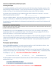### sonia cordero cardenas san andres university – la paz •jaime rojas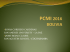### Review for the Final Exam (STA248)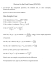### Homework 2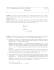### The Futurama Theorem.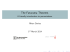### article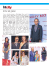### Thrust Force Analysis of a Rotating Ionocraft Under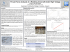### 7.1.18